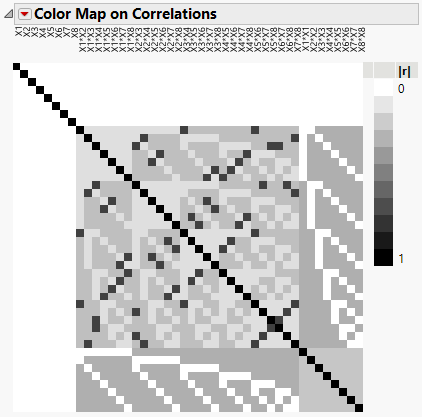Publication date: 08/13/2020

## Analysis of Experimental Data

In general, you want to fit a model that permits the possibility that two-way interactions are active. You also might want to include pure quadratic terms in your model. You might want to postulate a full second-order model, or you might want to specify an a priori model containing only certain second-order terms.

### Two-Way Interactions

In fitting such a model, you need to be mindful of two facts:

two-way interaction effects and quadratic effects are often correlated

two-way interaction and quadratic effects cannot all be estimated simultaneously

Figure 7.23 shows a Color Map on Correlations for the design with eight continuous factors shown in Figure 7.22. The color map is for a full quadratic design. The eight pure quadratic effects are listed to the far right. You can construct this plot by using DOE > Design Diagnostics > Evaluate Design and entering the appropriate terms into the Alias Terms list. See Alias Terms in the Evaluate Designs section.

Figure 7.23 Color Map on Correlations for Full Quadratic ModelUse your cursor to hold your mouse pointer over the cells of the color map in order to see the absolute correlations between effects. You see that main effects are uncorrelated with all two-way interaction and pure quadratic effects. You also see that none of the effects are completely confounded with other effects because the only red cells are on the main diagonal. But note that some of the absolute correlations between two-factor interactions are substantial, with some at 0.75. Note also that absolute correlations between two-factor interactions and pure quadratic effects are either 0 or 0.3118.

If only main and pure quadratic effects are active, you can fit a saturated model that contains main effects and quadratic effects. This model will result in effect estimates that are unbiased, assuming no active three-way or higher order effects.

Because of the correlations involving second-order effects, you must be careful in fitting a model with two-way interactions. Analysis methodologies include the following, where the first is preferred:

The method of Efficient Model Selection performs well, especially if many effects are likely to be active. See Effective Model Selection for DSDs in the The Fit Definitive Screening Platform section.

Forward stepwise or all possible subsets regression performs adequately if the following conditions hold:

The number of active effects is no more than half the number of runs.

There are at most two active two-way interactions or at most one active quadratic effect.

### Forward Stepwise Regression or All Possible Subsets Regression

This method consists of first specifying a full response surface model. Then do one of the following:

Use forward stepwise regression with the Stopping Rule set to Minimum AICc and the Rules set to Combine to ensure model heredity.

Use All Possible Models regression, where you select the option that imposes the heredity restriction and use the AICc criterion for model selection.

You cannot fit the full response surface model because the number of runs is less than the number of parameters. So your analysis depends on the assumption of effect sparsity, where you assume that the number of active effects is less than the number of runs. This approach has some limitations:

If the number of active effects exceeds half the number of runs, both stepwise and all possible models regression have difficulty finding the correct model.

The power of tests to detect moderate quadratic effects is low. A quadratic effect must exceed three times the error standard deviation for the power to exceed 0.9.

Because of effect confounding, several models might be equivalent. Additional runs will be necessary to resolve the confounding.